关于attention机制在nlp中的应用总结

attention 总结

Attention函数的本质可以被描述为一个查询（query）到一系列（键key-值value）对的映射，如下图。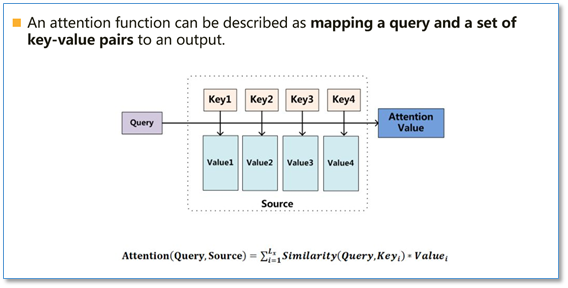• 第一步是将query和每个key进行相似度计算得到权重，常用的相似度函数有点积，拼接，感知机等；
• 第二步一般是使用一个softmax函数对这些权重进行归一化
• 最后将权重和相应的键值value进行加权求和得到最后的attention
• 目前在NLP研究中，key和value常常都是同一个，即key=value。
•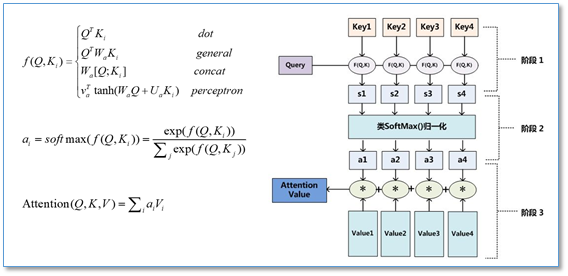• Attention在NLP中其实我觉得可以看成是一种自动加权，它可以把两个你想要联系起来的不同模块，通过加权的形式进行联系。目前主流的计算公式有以下几种：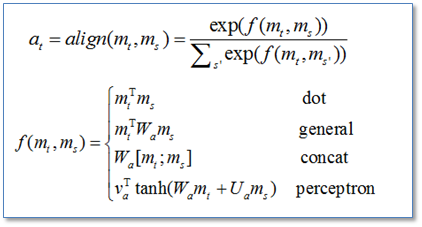通过设计一个函数将目标模块mt和源模块ms联系起来，然后通过一个soft函数将其归一化得到概率分布。

• 目前Attention在NLP中已经有广泛的应用。它有一个很大的优点就是可以可视化attention矩阵来告诉大家神经网络在进行任务时关注了哪些部分。不过在NLP中的attention机制和人类的attention机制还是有所区别，它基本还是需要计算所有要处理的对象，并额外用一个矩阵去存储其权重，其实增加了开销。而不是像人类一样可以忽略不想关注的部分，只去处理关注的部分。

一、传统encoder-decoder模型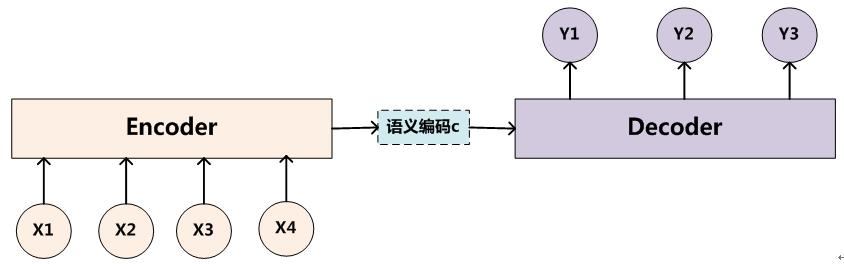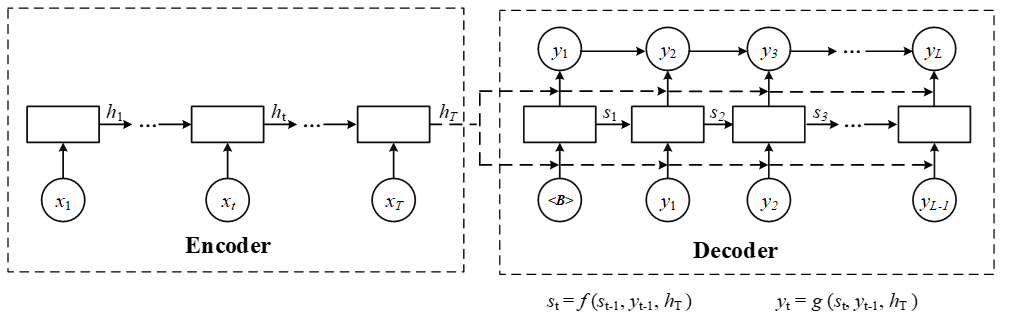1.1 encoder

• 对于输入序列$x=\left({x}_{1},...,{x}_{{T}_{x}}\right)$$x=(x_1,...,x_{T_x})$,其会将输入序列如图所示编码成一个context vector c ，encoder一般使用RNN，在RNN中，当前时间的隐藏状态是由上一时间的状态和当前时间输入决定的，也就是
${h}_{t}=f\left({x}_{t},{h}_{t-1}\right)$$h_t=f(x_t,h_{t-1})$
• 获得了各个时间段的隐藏层以后，再将隐藏层的信息汇总，生成最后的语义向量 c，相当于把整个句子的信息都包含了，可以看成整个句子的一个语义表示。
$c=q\left({h}_{t},...,{h}_{{T}_{x}}\right)$$c=q(h_t,...,h_{T_x})$

其中的f和q是非线性的函数
例如，在论文中有使用
$q\left({h}_{t},...,{h}_{{T}_{x}}\right)={h}_{{T}_{x}}$$q(h_t,...,h_{T_x}) =h_{T_x}$

来简化计算

1.2 decoder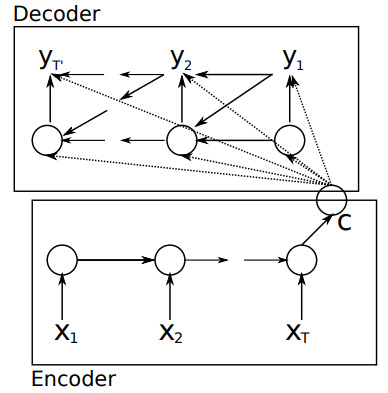$p\left(y\right)=\prod _{t=1}^{T}p\left({y}_{t}|\left\{{y}_{1},...,{y}_{t}\right\},c\right)$

• 在RNN中（如上图所示），t时刻隐藏状态${s}_{t}$$s_t$为：

${s}_{t}=f\left({s}_{t-1},{y}_{t-1},c\right)$$s_t =f(s_{t-1},y_{t-1},c)$

• 而联合条件分布为：

$p\left(y\right)=\prod _{t=1}^{T}p\left({y}_{t}|\left\{{y}_{1},...,{y}_{t}\right\},c\right)=q\left({y}_{t-1},{s}_{t},c\right)$

其中s是输出RNN中的隐藏层，C代表之前提过的语义向量，${y}_{t-1}$$y_{t−1}$表示上个时间段的输出，反过来作为这个时间段的输入。而q则可以是一个非线性的多层的神经网络，产生词典中各个词语属于${y}_{t}$$y_t$的概率。

1.3 存在问题：

“一个潜在的问题是，采用编码器-解码器结构的神经网络模型需要将输入序列中的必要信息表示为一个固定长度的向量，而当输入序列很长时则难以保留全部的必要信息（因为太多），尤其是当输入序列的长度比训练数据集中的更长时。”

二、加入attention

论文一：

NEURAL MACHINE TRANSLATION BY JOINTLY LEARNING TO ALIGN AND TRANSLATE

这篇论文中首次将attention用到了nlp领域，论文中提出了一个想法，目前机器翻译的瓶颈在于无论是多长的输入，大家的普遍做法都是将所有输入通过一些方法合并成一个固定长度的向量去表示这个句子，这会造成的问题是，如果句子很长，那么这样的方法去作为decoder的输入，效果并不会很好。

相比于之前的encoder-decoder模型，attention模型最大的区别就在于它不在要求编码器将所有输入信息都编码进一个固定长度的向量之中。相反，此时编码器需要将输入编码成一个向量的序列，而在解码的时候，每一步都会选择性的从向量序列中挑选一个子集进行进一步处理。这样，在产生每一个输出的时候，都能够做到充分利用输入序列携带的信息。而且这种方法在翻译任务中取得了非常不错的成果。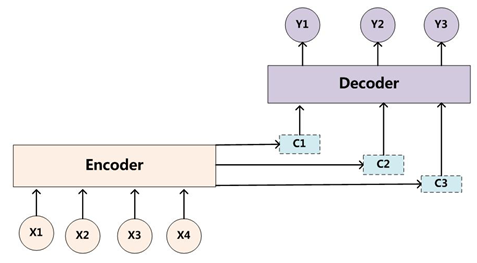1.1 编码（encoder）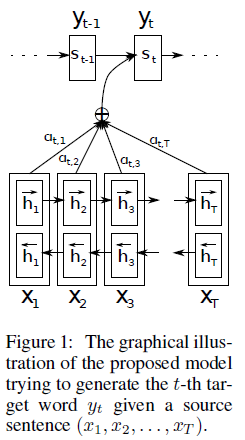1.2 解码（decoder）

1.2.1 context vector c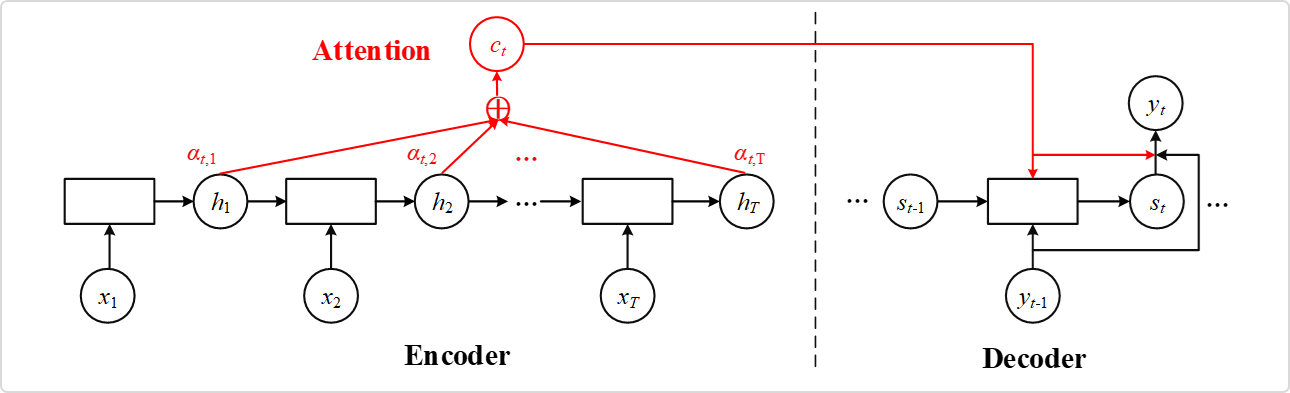${c}_{i}=\sum _{j=1}^{{T}_{x}}{a}_{ij}{h}_{ij}$$c_i=\sum_{j=1}^{T_x}a_{ij}h_{ij}$

${a}_{ij}=\frac{exp\left({e}_{ij}\right)}{\sum _{k=1}^{{T}_{x}}exp\left({e}_{ik}\right)}$$a_{ij}=\frac{exp(e_{ij})}{\sum^{T_x}_{k=1}exp(e_{ik})}$

${e}_{ij}=a\left({s}_{j-1},{h}_{i}\right)$$e_{ij}=a(s_{j-1},h_i)$

• 注：${h}_{j}$$h_j$为encoder的隐状态，${s}_{j}$$s_j$为decoder的隐状态
• ${a}_{ij}$$a_{ij}$的值越高，表示第i个输出在第j个输入上分配的注意力越多，在生成第i个输出的时候受第j个输入的影响也就越大。

• ${e}_{ij}$$e_{ij}$ ：encoder i处隐状态和decoder j-1 处的隐状态的匹配 match，此处的 alignment model a 是和其他神经网络一起去训练（即 joint learning），其反映了${h}_{j}$$h_j$的重要性

1.2.2 其余部分

$p\left(y\right)=\prod _{t=1}^{T}p\left({y}_{t}|\left\{{y}_{1},...,{y}_{t}\right\},{c}_{i}\right)=q\left({y}_{t-1},{s}_{t},{c}_{i}\right)$

• 在RNN中（如上图所示），t时刻隐藏状态${s}_{t}$$s_t$为：
${s}_{t}=f\left({s}_{t-1},{y}_{t-1},{c}_{i}\right)$$s_t =f(s_{t-1},y_{t-1},c_i)$

1.3 注意力矩阵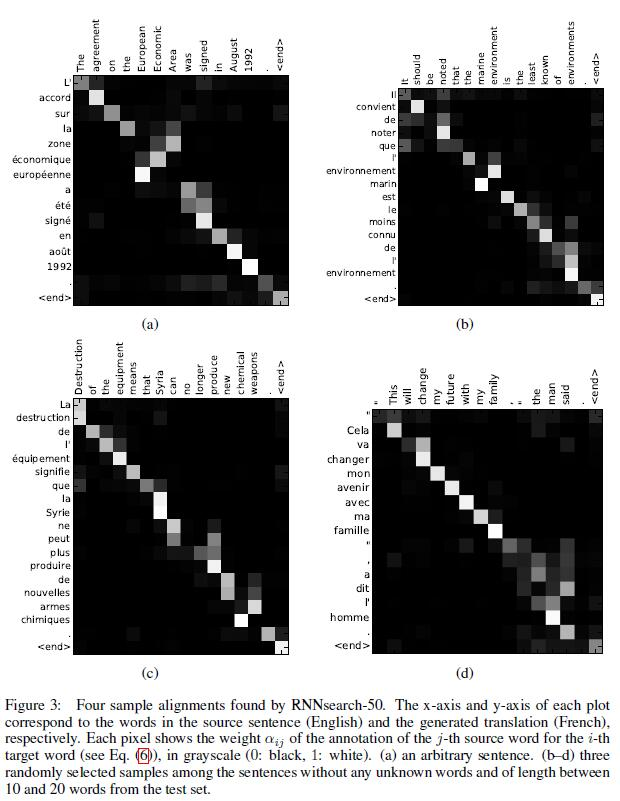论文二、

Effective Approaches to Attention-based Neural Machine Translation

• 1.global attention与论文一提到的模型很像，但做了简化
• 2.local attention是一种介于soft和hard attention之间的模型，他相对于global attention或者soft attention计算量更小，同时不像hard attention，local attention是可微分的
1.global attention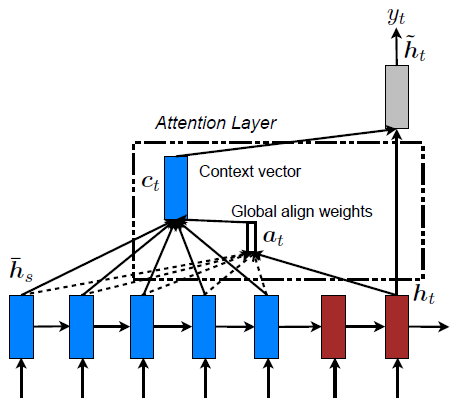{a}_{t}\left(s\right)=align\left({h}_{t},{h}_{s}\right)=\frac{exp\left(score\left({h}_{t},{h}_{s}\right)\right)}{\sum _{{s}^{\prime }}exp\left(score\left({h}_{t},{h}_{s}^{\prime }\right)\right)}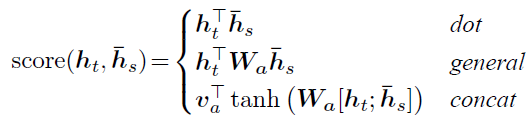${a}_{ij}=\frac{exp\left({e}_{ij}\right)}{\sum _{k=1}^{{T}_{x}}exp\left({e}_{ik}\right)}$$a_{ij}=\frac{exp(e_{ij})}{\sum^{T_x}_{k=1}exp(e_{ik})}$

${e}_{ij}=a\left({s}_{j-1},{h}_{i}\right)={v}_{a}^{T}tanh\left({W}_{a}{s}_{i-1}+{U}_{a}{h}_{j}\right)$$e_{ij}=a(s_{j-1},h_i)=v^T_atanh(W_as_{i-1}+U_ah_j)$

2.local attention

local attention是一种介于soft和hard之间的方式，使用了一个人工经验设定的参数D去选择一个以${p}_{t}$$p_t$为中心，$\left[{p}_{t}-D,{p}_{t}+D\right]$$[ p_t-D,p_t+D ]$为窗口的区域，进行对应向量的weighted sum，故不像global attention，local alignment vector ${a}_{t}$$a_t$的维数是固定的，不随输入序列长度变化而变化，它的维度固定为2D+1

• 1.单调对应(Monotonic alignment): 设定${p}_{t}=t$$p_t = t$
• 2.Predictive alignment:
• 使用${h}_{t}$$h_t$去预测${p}_{t}$$p_t$所在位置：
S为输入序列长度，此处保证了${p}_{t}$$p_t$一定落在输入序列内
${v}_{p}，{W}_{p}$$v_p，W_p$为权重矩阵
$p_t=S~*sigmoid(v_p^Ttanh(W_ph_t))$
• 为了使得最后的求和更加的偏好靠近位置${p}_{t}$$p_t$ 附近的向量，又在求和时加入了一个高斯函数，该函数u =${p}_{t}$$p_t$,使得${p}_{t}$$p_t$周围的向量得到的权重更大，(s即为一般公式中的x，${p}_{t}$$p_t$为u，${p}_{t}$$p_t$为高斯分布峰值)
{a}_{t}\left(s\right)=align\left({h}_{t},{h}_{s}^{\prime }\right)exp\left(-\frac{\left(s-{p}_{t}{\right)}^{2}}{2\sigma }\right)a_t(s)=align(h_t,h'_s)exp(-\frac{(s-p_t)^2}{2\sigma})

此处依据经验$\sigma =\frac{D}{2}$$\sigma = \frac{D}{2}$

3.模型具体实现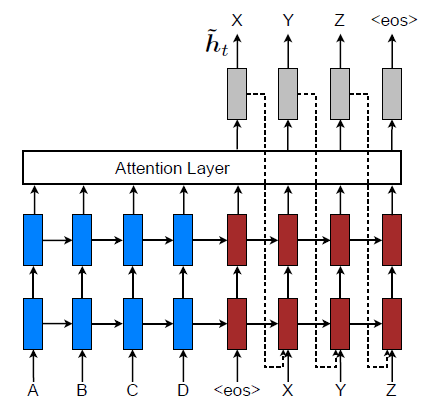${h}_{t}^{\prime }=tanh\left({W}_{c}\left[{c}_{t};{h}_{t}\right]\right)$$h'_t = tanh(W_c[c_t;h_t])$

$p\left({y}_{t}|{y}_{$p(y_t|y_{

• 论文一中使用的是${c}_{i}$$c_i$，其和上一个输出${y}_{i-1}$$y_{i-1}$,隐状态${s}_{i-1}$$s_{i-1}$一起作为时间t的输入
• 本文中使用${h}_{t}^{\prime }$$h'_t$作为输入，但是此处并未做对比试验比较两者的差别

4.结果比较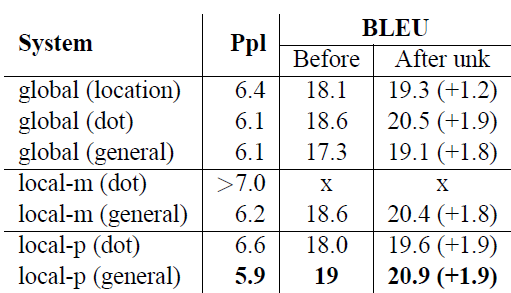4.补充

关于soft attention和hard attention

2.Hard Attention Model

Hard AM在图像里证明有用，但是估计在文本里面用处不大，因为这种单词一一对齐明显要求太高，如果对不齐对后续处理负面影响很大，所以你在NLP的文献里看不到用Hard AM的，估计大家都试过了，效果不好。

3.介于Soft 和Hard之间

Soft AM要求输入句子每个单词都要赋予单词对齐概率，Hard AM要求从输入句子中精确地找到一个单词来和输出单词对齐，那么可以放松Hard的条件，先找到Hard AM在输入句子中单词对齐的那个单词大致位置，然后以这个单词作为轴心，向左向右拓展出一个大小为D的窗口，在这个2D+1窗口内的单词内进行类似Soft AM的对齐概率计算即可。

论文三: ABCNN: Attention-Based Convolutional Neural Network for Modeling Sentence Pairs

• 参考资料：
卷积神经网络介绍
论文解读

• 这篇论文就提出了3中在CNN中使用attention的方法，是attention在CNN中较早的探索性工作。

• 传统的CNN在构建句对模型时如上图，通过每个单通道处理一个句子，然后学习句子表达，最后一起输入到分类器中。这样的模型在输入分类器前句对间是没有相互联系的，作者们就想通过设计attention机制将不同cnn通道的句对联系起来
• 这篇文章介绍的是利用带注意力机制的卷积神经网络进行句子对建模。句子对建模是自然语言处理中的一个经典问题，在诸如答案选择（Answer Selection, AS）、 释义鉴定（Paraphrase Identification, PI）、文本继承（Textual Entailment, TE）等场景中都有应用。
• 之前的相关工作多局限于：
• (1) 设计针对特定场景的特定系统；
• (2) 对每个句子分开考虑，很少考虑句子之间的影响；
• (3) 依赖人工设计的繁杂特征。
• 该模型主要贡献有：
• (1) 可以应对多种场景的句子对建模问题，泛化能力强；
• (2) 利用注意力机制，在建模时考虑句子之间的影响；

1.注意力机制

1.1 ABCNN-1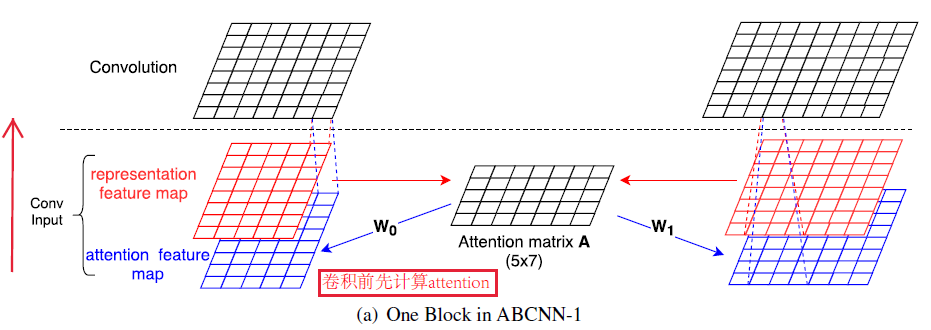1.2 ABCNN-2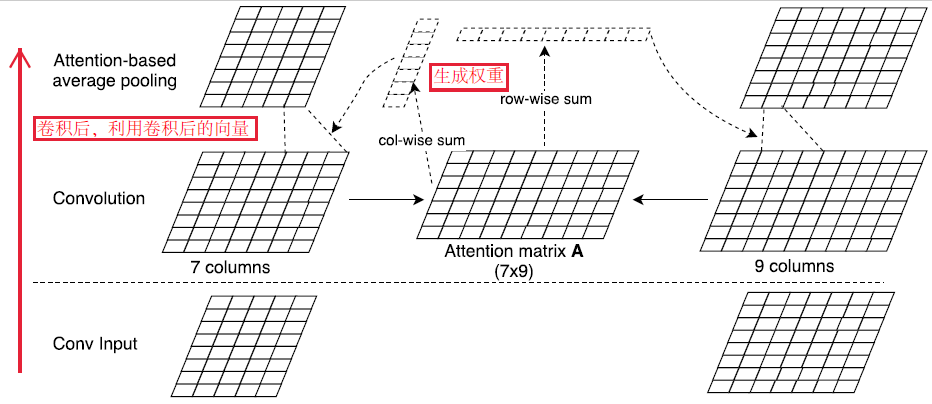在卷积后，对于卷积完成的两个矩阵，进行match，match后按照行和列的求和生成两个矩阵各自的权重，再使用权重对其进行

1.3 ABCNN-3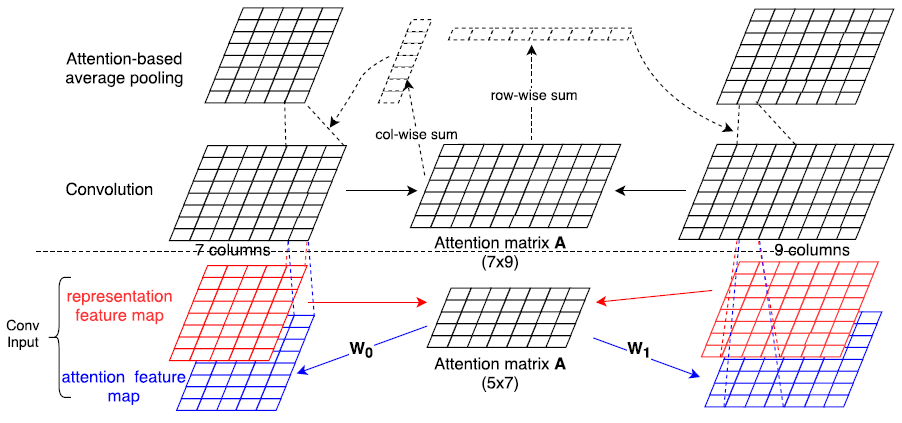论文四：attention is all you need

• 1.不同于以往主流机器翻译使用基于RNN的seq2seq模型框架，该论文用attention机制代替了RNN搭建了整个模型框架。